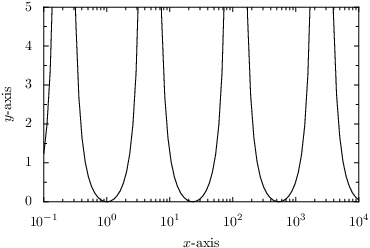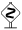# PyX — Example: graphs/axis.py

0.3 KB
60.6 KB
75.0 KB
37.0 KB
40.6 KB

## Using a logarithmic axis and defining the axis range and its label```from pyx import *

g = graph.graphxy(width=8,
x=graph.axis.log(min=1e-1, max=1e4, title=r"\$x\$-axis"),
y=graph.axis.lin(max=5, title=r"\$y\$-axis"))
g.plot(graph.data.function("y(x)=tan(log(1/x))**2"))
g.writeEPSfile("axis")
g.writePDFfile("axis")
g.writeSVGfile("axis")
```

### Description

Sometimes, you have to visualize rather pathological funtions containing divergencies and/or varying over a large parameter range. This is best done using an appropriate axis. Here, we show a simple example how to do this with PyX.

In order to change the properties of the graph axes, we have to create appropriate instances of an axis class and pass them as keyword arguments to the `graph.graphxy` constructor. A number of predefined axes is contained in the `graph.axis` module. Here, we use a linear (abbreviated as `graph.axis.lin`) and a logarithmic (abbreviated as `graph.axis.log`) axis. In both cases, we pass a minimal and maximal value for the axis range. Whereas for the x-axis this is not strictly necessary (we could as well tell the `graph.data.function` instance to emit data in a certain x-range), it is important for the y-axis because the function diverges for certain x values. If we do not set the y range manually, we would obtain a rather compressed display of the function in the y-direction, which, moreover, would strongly depend on the sampling points choosen by PyX along the x-direction. Since the function only diverges towards positive y-values, we only need to set the maximal value of the y-axis (using the `max`-argument). Of course, you can also fix the lower end of the axis range by providing a `min`-argument. In order to introduce the `title` keyword, we give names to the axes.Note how PyX changes the way the x-axis is drawn. Instead of simple decimal numbers, an exponential notation is used. This happens automatically by default, if at least one of the values to be shown becomes 10,000 or bigger smaller than 0.001. This behaviour can be altered by using a different axis texter.